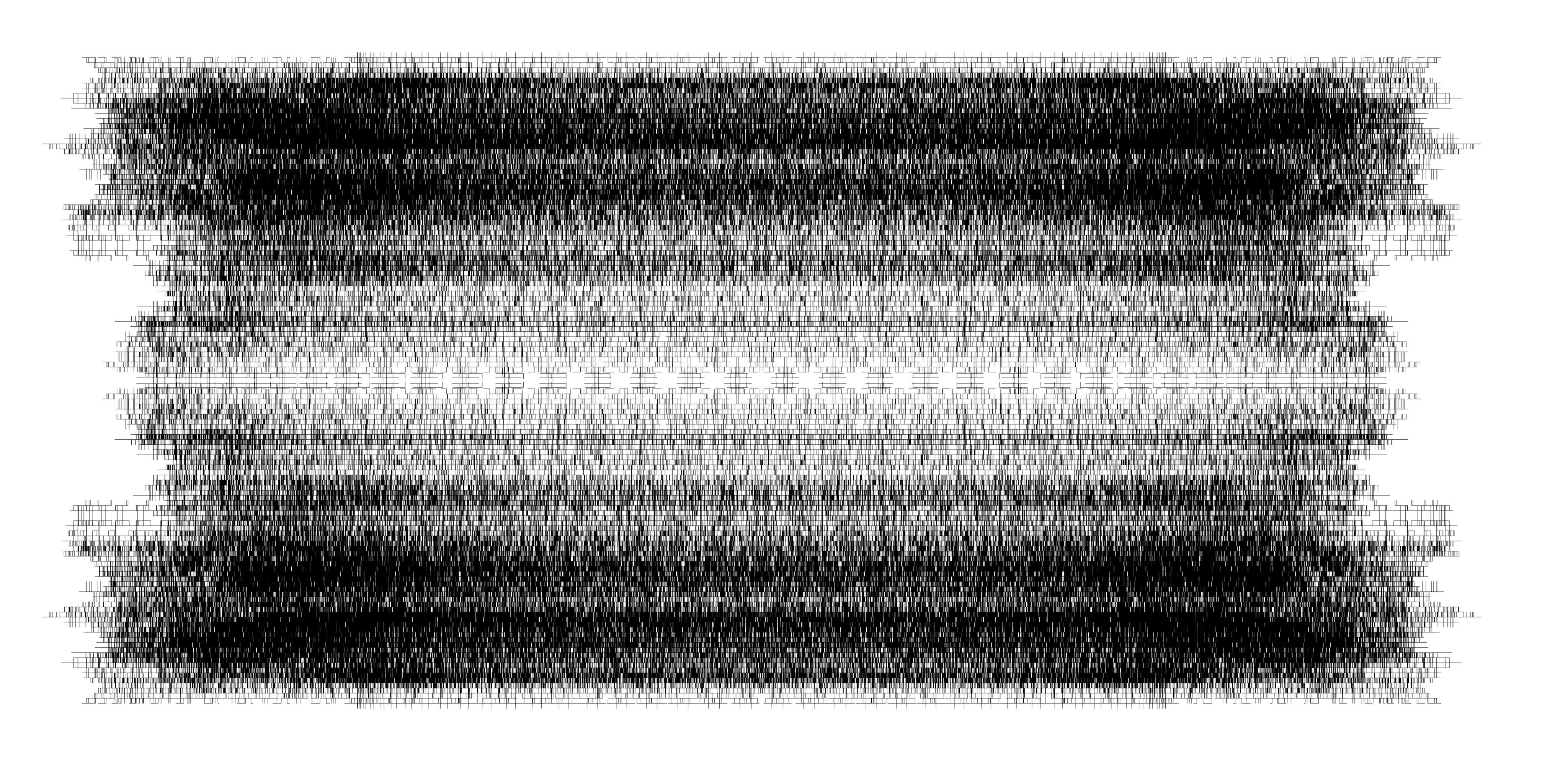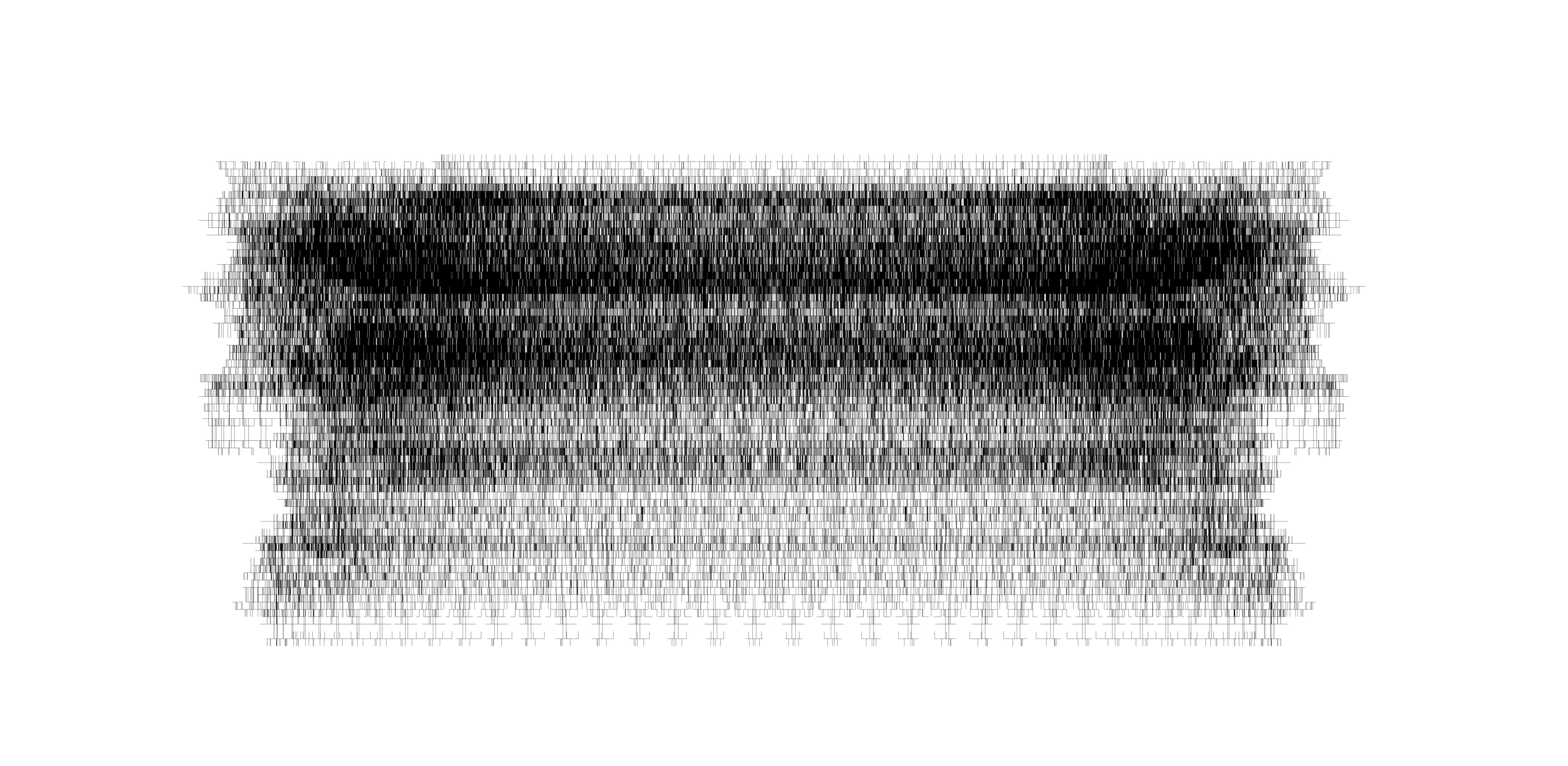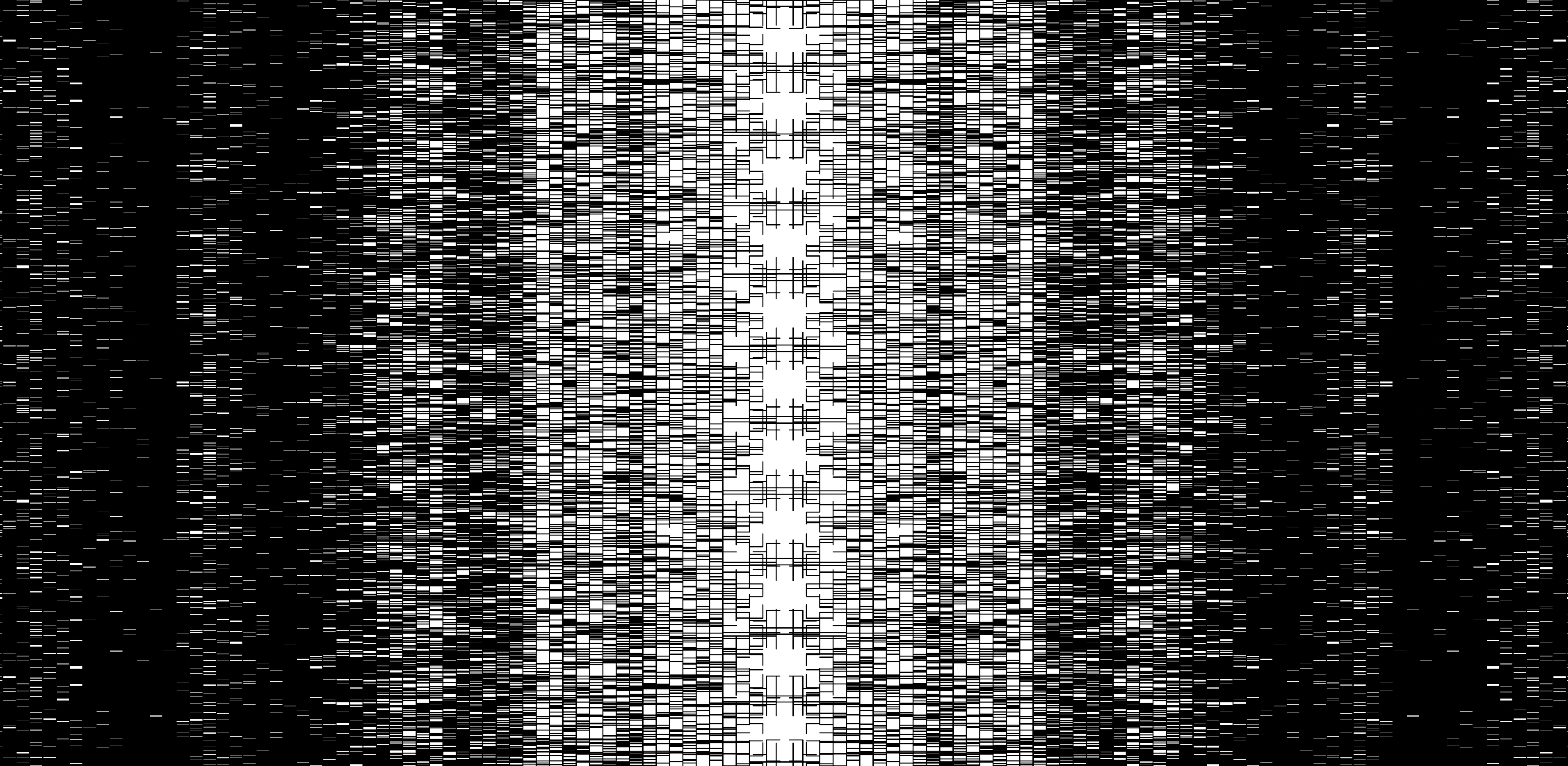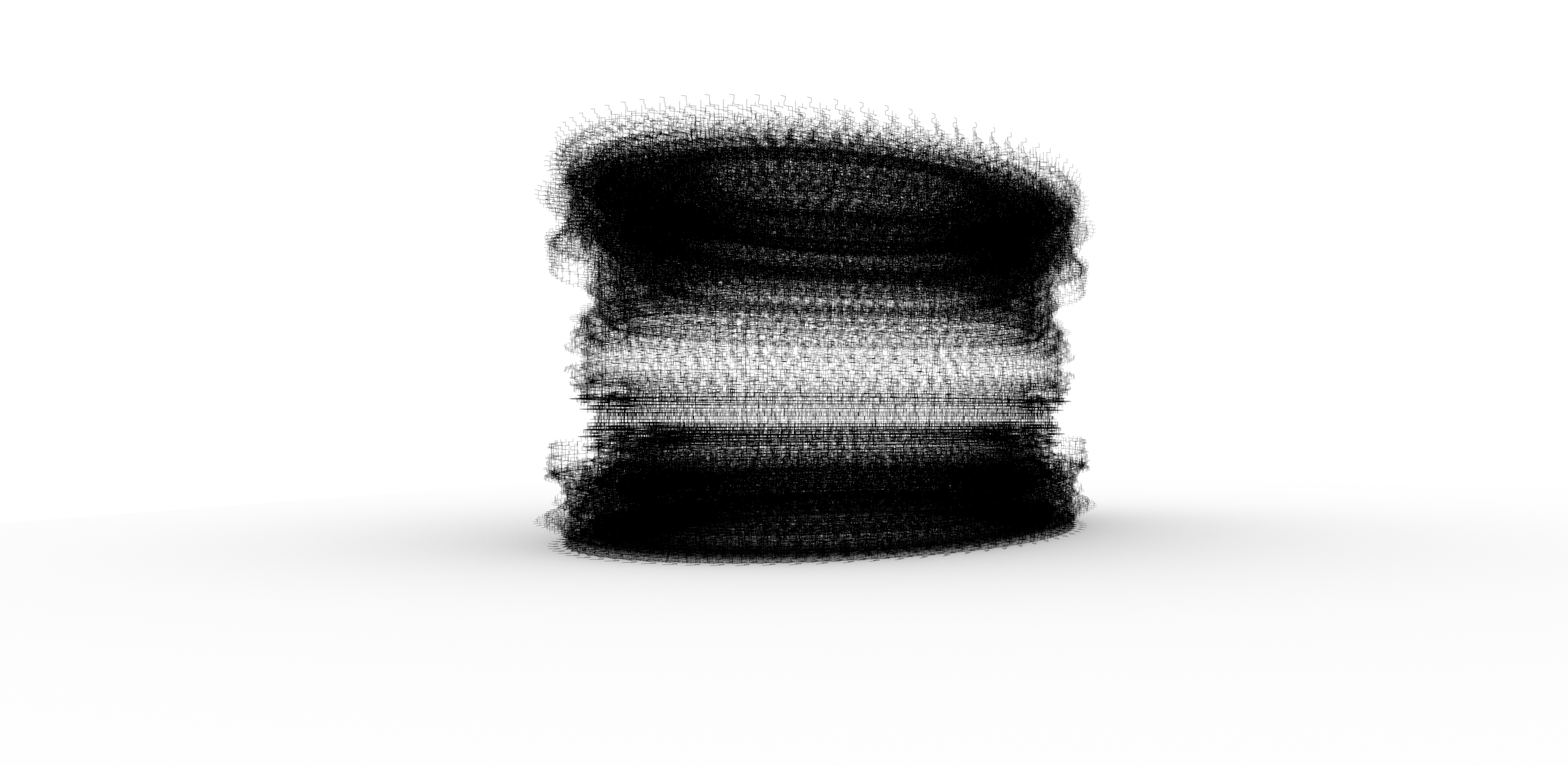## discrete computation | DATA simulation | Mathematics | 2018

This project computes new orders of algorithmic complexities, by transforming simulation based entropic energy into information and vice-versa. It calculates a random data-set, a point cloud with Cartesian X, Y, and Z values. During the computation, the depth values are usurped while the remaining X, Y values are utilised to compute and simulate a two-dimensional wall of data that is systematically synthesised, then divided arithmetically into smaller parts. Until all the data within the archive neutralises or reveals the incompleteness of the axioms on which the algorithmic operations were based in the first place, thus opening onto incomputable probabilities in discrete states. The results define the limits of a computable discrete quantity, increasing its resolution by branching and converging, yet constrained to avoid other elements in its binary expansion. All suspended on an algorithmic sequence by transforming the new data growth into discrete units. The result is arrayed three-dimensionally in the simulation.